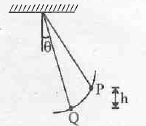#### Thank you for registering.

One of our academic counsellors will contact you within 1 working day.

Click to Chat

1800-5470-145

+91 7353221155

CART 0

• 0
MY CART (5)

Use Coupon: CART20 and get 20% off on all online Study Material

ITEM
DETAILS
MRP
DISCOUNT
FINAL PRICE
Total Price: Rs.

There are no items in this cart.
Continue Shopping

# Q1 The bob of a simple pendulum of length I is released from point P. What is the angle made by the net acceleration of the bob with the string at point Q.8 years ago

Dear Anurag,

 A typical simple pendulum consists of a heavy pendulum bob (mass = M) suspended from a light string. It is generally assumed that the mass of the string is negligible. If the bob is pulled away from the vertical with some angle, q, and released so that the pendulum swings within a vertical plane, the period of the pendulum is given as:   T = 2p (L/g)1/2(1 + 1/4 sin2q/2 + 9/16sin4q/2 + ...)            Equation 1   where L is the length of the pendulum and g is the acceleration due to earth''s gravity. Note only the first three terms in the infinite series isgiven in the above equation. The period is defined as the time required for the pendulum to complete one oscillation. That is, if the pendulum is released at some point, P, the period is defined as the time required for the pendulum to swing along its path and return to point P.

The above formula for the pendulum''s period is greatly simplified if we limit the initial angle q to small values. If q is small, we can approximate the period of the pendulum with the following expression.

T = 2(L/g)1/2         Equation 2

Note that the period in this expression is independent of the pendulum''s mass as initial angle, q. It is important to understand that the above equation is valid only in the small angle approximationIf the magnitude of the displacement is sufficiently small, and if we neglet the effects of friction and air resistance, the motion of the mass is relatively simple and is called simple harmonic motion.

Cracking IIT just got more exciting,It s not just all about getting assistance from IITians, alongside Target Achievement and Rewards play an important role. ASKIITIANS has it all for you, wherein you get assistance only from IITians for your preparation and win by answering queries in the discussion forums. Reward points 5 + 15 for all those who upload their pic and download the ASKIITIANS Toolbar, just a simple  to download the toolbar….

So start the brain storming…. become a leader with Elite Expert League ASKIITIANS

Thanks

Aman Bansal